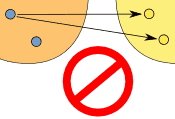# Functions

Standard

## A Function is Special

A function is a type of equation.

A function has special rules it must follow:

In a function there can ONLY be ONE output(y) for every input (x).

Just like I stated before, a Function is Special! 🙂

• It must work for every possible input value
• And it has only one relationship for each input valueTHIS IS A FUNCTION!                  THIS IS NOT A FUNCTION!

### Example: y = x²

FUNCTIONCould also be written as a table:

X: x Y: x2
3 9
1 1
0 0
4 16
-4 16

It is a function, because:

• Every element in X is related to Y
• No element in X has two or more relationships
• The X values DO NOT repeat

So it follows the rules.

(Notice how both 4 and -4 relate to 16, which is allowed since 4 and -4 are two different numbers.)

### Example: This relationship is not a function:

NOT A FUNCTIONThis is not a function, for these reasons:

• Value “3” in X has no Y
• Value “4” in X has no Y
• Value “5” is related to more than one value in Y
• REMEMBER THE X VALUE CANNOT REPEAT